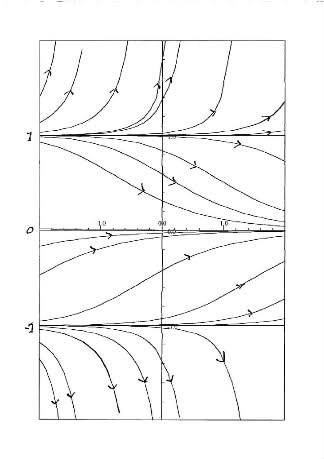# How to find the steady state solution

consider and determine the steady state solution of the differential equation below.

dy/dx = y(y-1)(y+1)

We can separate the variables, break the integrand into partial fractions, and integrate the fractions easily.

Solving gives y = the square root of 1 / (1 - e^(2t)).

as t goes to infinity, y goes to zero which the steady state solution.

But, the actual wording of the problem goes like this.

Find the steady state solution of the differential equation WITHOUT determining the exact solution and taking t to infinity.

Mute
Homework Helper
When the system reaches the steady state solution, what do you know about y(x) (in general)? How does the steady-state solution change as x changes? (hint: that's a trick question). Think about what that means in terms of your DE.

To find the steady state solution, I set dy/dx=0 in the differential equation

dy/dx=y(y-1)(y+1)

So, y=0, y=1 or y=-1.

The book says the only answer is y=0. Why are y=-1 and y=+1 rejected as steady state solutions?

epenguin
Homework Helper
Gold Member
You give us an equation in y for dy/dx and a solution in terms of t.

Quite often when students give us answers we are able to work out what the questions were but generally we find it easier the other way round.Steady state may be - guessing - dy/dt = 0, and dx/dt = 0? Maybe your other solutions do not work for that? Like dy/dx = 0 , when dy/dt = 0 but dx/dt ≠ = 0, which is possible but not a steady state. ?

Nor really checked but doubtful about the solution you have given.

There is a typo in the solution that I write down on the posting.

It should be given in terms of x NOT t. sorry about that.

ie, y = the square root of 1/(1-e^(2x))

Back to my issue.

By setting dy/dx = 0, we should get three steady state solutions. y=0, y=1 or y=-1

Is there a way to verify that ONLY y=0 is correct which is the book's answer? Thanks.

epenguin
Homework Helper
Gold Member
OK, so not what I thought.

I trust you have redifferentiated your solution to check it is correct. I did and agree it is - but it is only one solution, not the general solution. You have forgotten to put in a constant of integration. I think the solution is

y = (1 - Ke^2x)^-(1/2)

with K arbitrary constant.

Then you can get solutions through any point in the x,y plane.

Including through those y values you mentioned. I will put up a plot of the solutions if I can and you will see they are just what you can expect qualitatively directly from the d.e.

Just from the language used, "steady state" really suggests x represents time. In that case I see nothing wrong with what you say and all three y's correspond to steady states. Just that y = 0 is a stable steady state to which anything starting between y = -1 and y = 1 converges, while the other two are unstable steady states from which anything off those lines however slightly goes away from them as time goes on. But they are called steady states nonetheless.

I don't know what trick Mute has in mind. Are you sure you reproduced the question verbatim?

Worry about the fact that some values of K in the solution, as also in your original solution, seem to give you infinite y.

Last edited:
epenguin
Homework Helper
Gold MemberWhat I mean. x → , y ↑